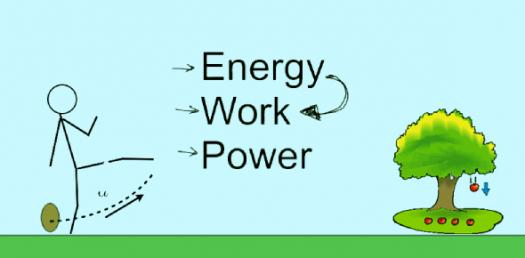# Test Your Knowledge About Work, Power And Energy! Trivia Questions Quiz

18 Questions | Attempts: 571
ShareSettingsTest Your Knowledge about Work, Power, and Energy using this Trivia Questions Quiz. Energy is the ability to do work, and power is the rate at which work is done. Do you know some of the factors that affect the rate of work? The quiz below is perfect for seeing how true this is. Do give it a shot and keep a lookout for others like it!

• 1.
If a force moves an object or changes its direction, what is being done?
• A.

Work

• B.

Power

• C.

Force

• D.

Motion

• 2.
The unit used to measure Work is:
• A.

Watt

• B.

Ampere

• C.

Newton

• D.

Joule

• 3.
The operation used  for calculating Work is:
• A.

• B.

Multiplication

• C.

Division

• D.

Subtraction

• 4.
Who does more work - a man who lifts a large box from the ground up into the back of a truck, or a man who puts the same box on a trolley and wheels it up a ramp into the truck?
• A.

The man with the trolley

• B.

The man who lifts it

• C.

Both of them do the same work

• D.

Neither the man with the trolley nor the one who lifts it

• 5.
A weightlifter lifts barbells of 200 Newtons above his head to a height of 2 meters. How much work does he do?
• A.

100 N

• B.

100 N-m

• C.

400 N

• D.

400 N-m

• 6.
Energy and work use the same unit of the Joule because:
• A.

That is the unit chosen by the scientist named Pascal who studied heat

• B.

Energy is required to do work

• C.

Both measure the speed at which power is used

• D.

Both concepts use force as a variable

• 7.
One joule is equal to:
• A.

1 N-m

• B.

1 N/m

• C.

1 N

• D.

1 N/s

• 8.
Aside from the work is done, what another factor shall be considered in determining the power?
• A.

Distance

• B.

Time

• C.

Speed

• D.

Force

• 9.
The rate at which work is done is called ____________ .
• A.

Force

• B.

Work

• C.

Power

• D.

Energy

• 10.
Power is measured in:
• A.

Joule

• B.

N/s

• C.

Watt

• D.

Watt/s

• 11.
The old imperial unit for power is:
• A.

Calorie

• B.

Kilojoule

• C.

Horsepower

• D.

Watt

• 12.
If a weightlifter lifts 2000 Newtons to a height of 2 meters in 4 seconds, how powerful is he?
• A.

1000 N-m

• B.

1000 J/s

• C.

1000 W/s

• D.

1000 J

• 13.
To say that work is scientifically done, the force must make the object _________.
• A.

Change direction

• B.

Change position

• C.

Change mass

• D.

Change work

• 14.
A man exerts a force of 60 N to a table which causes it to move by 2.5 meters. What was the work done?
• A.

24 N-m

• B.

24 J

• C.

150 N-m

• D.

150 W

• 15.
What power is required for a person to do a 780 J in 45 s?
• A.

1.73 W

• B.

17.33 W

• C.

173.33 W

• D.

1733.33 W

• 16.
Suppose a box is lifted with a force of 97 N to a height of 5 meters, what could be the power used?
• A.

485 J

• B.

485 W

• C.

No power was used

• D.

Cannot be determined

• 17.
What is the force applied if the work done on a push cart is 350 J that covered a distance of 22 meters?
• A.

15.91 N

• B.

159.01 N

• C.

770 N

• D.

7700 N

• 18.
On what month is the annual Earth Day celebrated?
• A.

January

• B.

February

• C.

March

• D.

April

## Related TopicsBack to top
×

Wait!
Here's an interesting quiz for you.